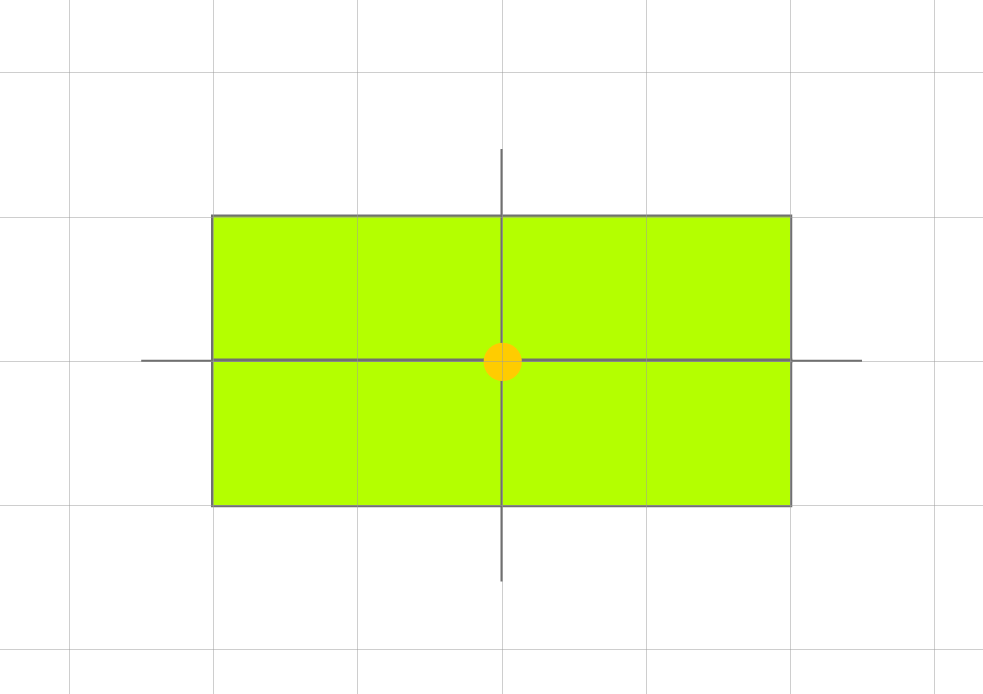Hey there! I’m currently working with matrices and grids to make a grid placement system. However I have run into a problem.

The way my system works, is that it takes in mouse target, it finds the nearest grid position to that mouse target. This works, however this only should happen for ‘plots’ with even length and even breadth.
The problem arises when you use odd lengths and/or odd breadth plots, because the center of that plot, does not lie on the grid lines, instead, it lies exactly between them.

Below is an explanation.Here, the plot is 2x4. As you can see, the center lies on the grid perfectly, and it’s position can be rounded to 4 to position the whole plot properly in a 4x4 stud grid.

Let us now consider an anomaly.

Here, the plot is a 2x3, which has even rows and odd columns. As you can see, the center does lie on the x-axis, but it does not lie on the y axis of the 4x4 stud grid.

So here, I can’t just do

``````function Snap(vec)
local newX = math.floor((vec.X / 4) + 0.5) * 4
local newY = math.floor((vec.Y / 4) + 0.5) * 4
return Vector3.new(newX, 0 newY)
end
``````

Because the above code only works for even x even plots. If I did apply it for even x odd plots, the following would be the result.Here, the center lies on the 4x4 grid, but it’s tiles are out of place.

I wanted to know how I can offset my snap function to handle odd dimensions. I have a matrix for the even x odd plot, which looks like this:

``````plot = {
{0,0,0},
{0,0,0}
}
``````

I’m not sure if the matrix will help, I just wanted to fix my snap function. If you guys have any ideas, please let me know!